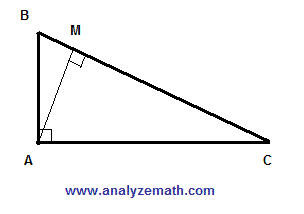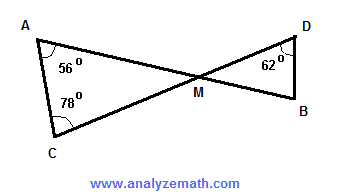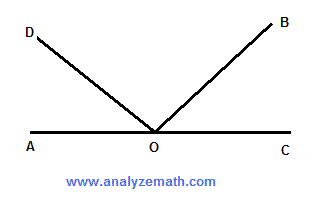Grade 9 geometry problems and questions with answers are presented. These problems deal with finding the areas and perimeters of triangles, rectangles, parallelograms, squares and other shapes. Several problems on finding angles are also included. Some of these problems are challenging and need a good understanding of the problem before attempting to find a solution. Also Solutions and detailed explanations are included.

1. Angles A and B are complementary and the measure of angle A is twice the measure of angle B. Find the measures of angles A and B,

2. ABCD is a parallelogram such that AB is parallel to DC and DA parallel to CB. The length of side AB is 20 cm. E is a point between A and B such that the length of AE is 3 cm. F is a point between points D and C. Find the length of DF such that the segment EF divide the parallelogram in two regions with equal areas..

3. Find the measure of angle A in the figure below..

4. ABC is a right triangle. AM is perpendicular to BC. The size of angle ABC is equal to 55 degrees. Find the size of angle MAC..

5. Find the size of angle MBD in the figure below..

6. The size of angle AOB is equal to 132 degrees and the size of angle COD is equal to 141 degrees. Find the size of angle DOB..

7. Find the size of angle x in the figure..

8. The rectangle below is made up of 12 congruent (same size) squares. Find the perimeter of the rectangle if the area of the rectangle is equal to 432 square cm..

9. ABC is a right triangle with the size of angle ACB equal to 74 degrees. The lengths of the sides AM, MQ and QP are all equal. Find the measure of angle QPB..

10. Find the area of the given shape..

11. Find the area of the shaded region..

12. The vertices of the inscribed (inside) square bisect the sides of the second (outside) square. Find the ratio of the area of the outside square to the area of the inscribed square..

Solutions and detailed explanations are included.

## Answers to the Above Questions

1. measure of A = 60 degrees, measure of B = 30 degrees
2. length of DF = 17 cm
3. measure of A = 87 degrees
4. size of angle MAC = 55 degrees
5. size of angle MBD = 72 degrees
6. size of angle DOB = 93 degrees
7. size of angle x = 24 degrees
8. perimeter of large rectangle = 84 cm
9. measure of angle QPB = 148 degrees
10. area of given shape = 270 square cm
11. area of shaded region = 208 square cm
12. ratio of area of outside square to area of inscribed square = 2:1